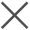• NAS性能测试

最近更新时间：2019-01-14 09:44:43

您可以使用 FIO 进行吞吐和 IOPS 的性能测试。

性能测试前，请注意以下事项：

• 确认sunrpc_slot设置正确，详情请参考常见问题文档说明。
• 吞吐最大不会超过 ECS 带宽。如果您的 ECS 带宽只有 1Gbps，则吞吐最大可达到 125MB/s。

如下提供一些通用的性能测试样例。

说明 以下提供的预估值均为单台 ECS 测试的结果。要达到文件存储 NAS 官网性能指标，建议使用多台 ECS 进行测试。

随机读 IOPS 设置

fio -numjobs=1 -iodepth=128 -direct=1 -ioengine=libaio -sync=1 -rw=randread -bs=4K -size=1G -time_based -runtime=60 -name=Fio -directory=/mnt单机测试值：22.1k

随机写 IOPS 设置

fio -numjobs=1 -iodepth=128 -direct=1 -ioengine=libaio -sync=1 -rw=randwrite -bs=4K -size=1G -time_based -runtime=60 -name=Fio -directory=/mnt单机测试值：13.4k

随机读吞吐

fio -numjobs=1 -iodepth=128 -direct=1 -ioengine=libaio -sync=1 -rw=randread -bs=1M -size=1G -time_based -runtime=60 -name=Fio -directory=/mntSSD性能型单机测试值：285MB

随机写吞吐

fio -numjobs=1 -iodepth=128 -direct=1 -ioengine=libaio -sync=1 -rw=randwrite -bs=1M -size=1G -time_based -runtime=60 -name=Fio -directory=/mntSSD性能型单机测试值：508MB

以上内容是否对您有帮助？
•